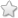# MHSB - 5 - Maths :: Multiplication and division

## Course Content / Subtopic list:

1. Multiply Given Two Numbers -> 3 Digit By 2 Digit
2. Multiply 3 Digit By 3 Digit, 4 Digit By 2 Digit
3. Divide Given Number By Another -> 4 Digit By 1 Dig
4. Divide 4 Digit By 2 Number

## Lesson Plan

[Contributor - Kripa S]

Objective: To revise multiplication and division of numbers

Subtopics to be covered:

- Multiply given two numbers -> 3 digit by 2 digit, 3 digit by 3 digit, 4 digit by 2 digit

- Divide given number by another -> 4 digit by 1 digit, 4 digit by 2 number

- Solve word problems based on multiplication and division

Learning Outcome:

- Students can multiply and divide given two numbers as in above categories

## Videos

[Contributor - Kripa S]

## Images

[Contributor - Kripa S]

## Activities

[Contributor - Kripa S]

## Transliteration

[Contributor - Satish Kothari]

 Term Transliterated Term Translated Term Multiplicand मल्टिप्लिकेन्ड गुण्य Multiplier मल्टीप्लायर गुणक Multiplication मल्टिप्लिकेशन गुणाकार Dividend डिविडेंड भाज्य Divisor डिवीज़र भाजक Division डिवीज़न भागाकार

## Worksheets

[Contributor - Ashwini Deshpande]

Worksheets [Version 2021]

## Assessments

[Contributor - Abhishek Bhatewara](0 votes)